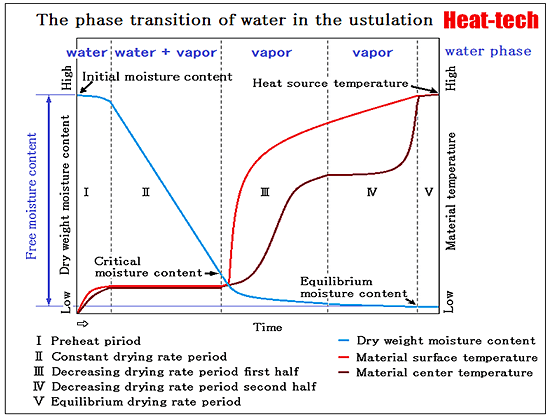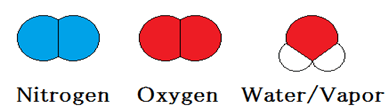# 3-1.Heat transfer and Mass transferIn the classification of thermodynamics, a dry process is a “The open system.”
Dryness is “evaporating and separating a liquid from a solid.”
Heat and pressure are operated, water is evaporated and it moves.
The height of heat is the intensity (Strength) of movement of the molecule which constitutes a substance.
The size of pressure is the number of molecules, and the intensity of a molecular movement.The dry process is typical “Three-body problem” of the thermodynamics for air = (nitrogen + oxygen) and vapor.
Three-body problem is “If the substance to deal with will be three or more pieces, a mathematical exact solution will not be obtained any longer”
It is defined at all that an exact solution is not calculated, therefor it is necessary to tackle with the accuracy of the practical use range.

Water has been held inside or material surface with a force such as the following.

Adhesion surface water → Interfacial tension
Capillary cord-like water → Capillary suction pressure
Capillary water suspended → Interfacial tension
Osmotic water → Osmotic suction force
Bound water → Affinity

The evaporated by moving the surface of the material the liquid by adding heat and pressure to the force.
Or, it is vaporized within the material, and then release it to move on the surface of the material.

The power used for transfer of heat
* Thermal conduction

The power used for movement of water or vapor
* Diffusion

The power used for evaporation
* Latent heat

The Heat transfer occur from the surface to the inside.
The movement of moisture occur to the surface from the inside
It acts in the opposite direction.

The most common in the drying process, it is heated and dried.
Wind drying which dried under reduced pressure to a surface by applying the wind is also common.
After carrying out a cooling freeze, it decompresses and vacuum drying to dry is also increasing gradually.

*The phase equilibrium of water and vapor

In a state in which vapor and water coexist in the closed space, evaporation of water stops when it reaches a concentration equilibrium constant.

Vapor contained in the water at normal temperature and pressure (25 ℃ · 1atm) is

Free energy of formation of water = -237.1KJ/mol
Free energy of formation of vapor = -228KJ/mol

H2O(liquid)→H2O(gas)+8.5kJ/mol at25℃・1atm

Concentration equilibrium constant of water of 25 ℃

Kc=[H2O(gas)]/[H2O(water)]=exp(-ΔG/RT)=exp(-8500/8.31×298)=0.03 at25℃・1atm

* 8500=8.5kJ/mol
* 298=K=273℃+25℃

∴ 3% vapor /97% water equilibrium state

Vapor contained in the water 60 ℃ at atmospheric pressure (60 ℃ · 1atm) is

Free energy of formation of water = -237.1KJ/mol
Free energy of formation of vapor = -228KJ/mol

Specific heat at constant pressure 75.3J / K · mol of water of 60 ℃
Specific heat at constant pressure 33.6J / K · mol of vapor of 60 ℃

ΔG(333)=8500+(-41.7)(333-298)-333(41.7)In333/298=8.5825kJ/mol

Concentration equilibrium constant of water of 60 ℃

Kc=[H2O(gas)]/[H2O(water)]=exp(-ΔG/RT)=exp(-858285/8.31×333)=0.045 at60℃・1atm

* 8582.5=8.5825kJ/mol
* 333=K=273℃+60℃

∴ 95.5% water / 4.5% vapor equilibrium state

Although it is in agreement with an experience value every day, if temperature is raised to 60℃, and based on 25℃, it will become easy to become vapor 1.5 times.